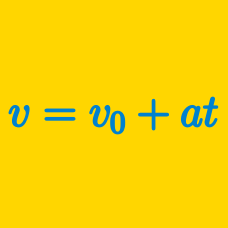Classical Mechanics

# Velocity vs. Acceleration

At time zero, a green car and a red car start from rest on a straight road from the same position in adjacent lanes. The green car moves with a constant speed of $$21 \text{ m/s}$$ and the red car accelerates uniformly at a rate of $$1.5 \text{ m/s}^2 .$$ Which car is ahead $$10$$ seconds later?

A car initially at rest starts to travel on a straight road. The car starts moving with a constant acceleration of $$12 \text{ m/s}^2.$$ After accelerating for $$4$$ seconds, the driver applies the brakes, and the car decelerates at a constant rate of $$-6 \text{ m/s}^2 .$$ From the point the car's brakes are applied, how long does it take for the car to come to a complete stop?

A body rises vertically up to a height of $$45 \text{ m}$$ in $$3$$ seconds, then falls back to the point of projection, with a constant acceleration during its entire movement. Assuming that the air resistance is negligible, is the following statement true or false?

"During its entire movement, the acceleration of the body is $$6 \text{ m/s}^2,$$ and its average velocity is $$25 \text{ m/s}.$$"

Cars $$A$$ and $$B$$ are moving in the same direction in adjacent straight lanes. If car $$A$$ has a bigger acceleration than car $$B,$$ does it mean that car $$A$$ is faster than car $$B?$$

Something that is accelerating is $$\text{_________}.$$

×

Problem Loading...

Note Loading...

Set Loading...